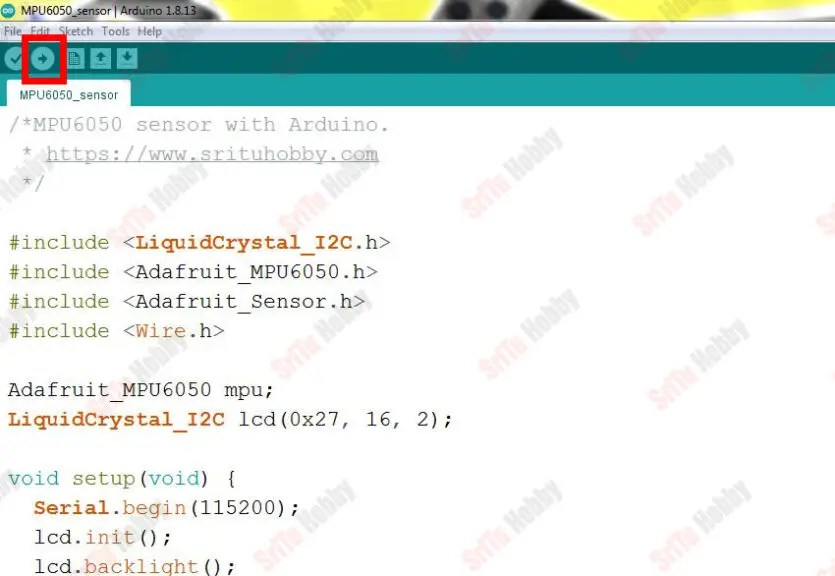# How to use MPU6050 with Arduino | Step by step instructions

## How to use MPU6050 with Arduino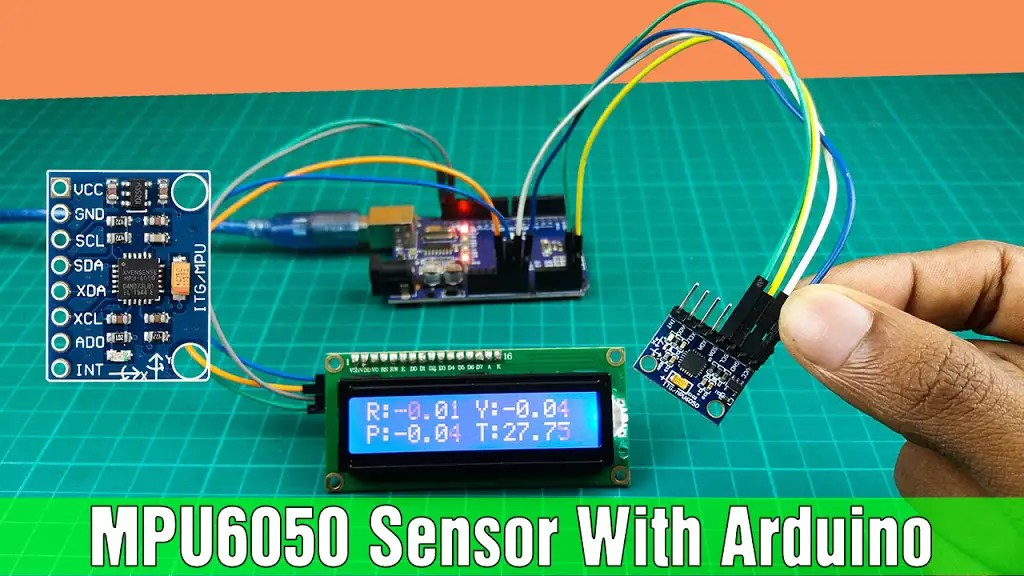Hello, welcome back. In this tutorial, I will show you how to use MPU6050 with Arduino. Through this sensor, we can measure rotation mainly on 6 axes. That is three axes through the accelerometer and three axes through the gyroscope. That is, this sensor includes both an accelerometer and a gyroscope. The MPU6050 sensor can also measure the static acceleration due to gravity, motion due to vibration, dynamic acceleration, and temperature. Also, the technology in this sensor is incorporated into anti-gravity activation systems. That includes things like helicopters, airplanes, space shuttles. We can make Self-balancing robots, drones, airplanes, etc using this MPU6050 sensor. So I hope the knowledge in this tutorial will be more useful to you.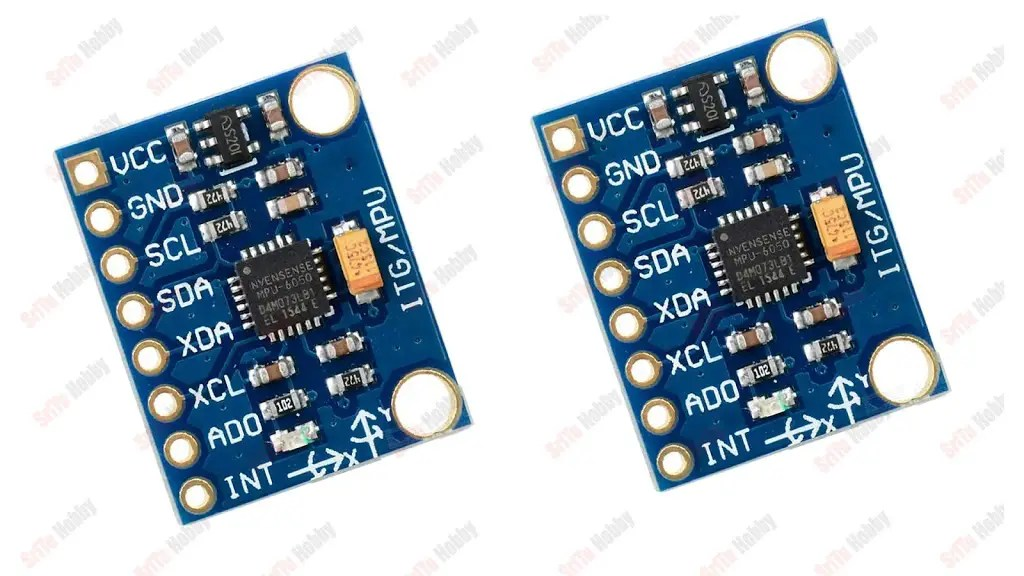### <br /> (adsbygoogle = window.adsbygoogle || []).push({});<br /> What is the MPU6050 sensor?

This type of sensor is made up of a system called MEMS (Micro-Electro-Mechanical-Systems). This includes both the accelerometer and the gyroscope.

#### What is the MEMS?

MEMS is simply called Micro-Electro-Mechanical-Systems. It is also a very small type of system created by micro components. These are made of materials such as silicon, polymers, and metals. It is also equipped with a microcontroller.

#### How to work an accelerometer?This also includes MEMS technology. What happens here is that the angle of inclination along the three axes X, y, and Z is identified. Also, the acceleration is calculated by measuring the difference in capacitance. This is designed on a silicon wafer. It also includes a mass that allows the polysilicon spring to travel through the outer plates. Therefore, when the acceleration is applied in a specific direction, there is a difference in capacitance between the plate and the mass. This value corresponds to an acceleration value. Also, the sensor converts this current difference into an analog voltage. The full-scale range of acceleration are +/- 2g, +/- 4g, +/- 8g, +/- 16g.

#### How to work gyroscope?This also includes MEMS technology. This allows us to identify the rotational velocity along with the X, Y, and X-axes. It is made up of four parts that react to the Coriolis effect. These move each other. When applied at an external angular ratio, the flexible part of the mass moves and displaces vertically. Also, the angle of the gyroscope is measured using the Coriolis effect. The full-scale range of output are +/- 250, +/- 500, +/- 1000, +/- 2000.
This external angular ratio is applied to three methods.
1.Roll- Here it is called roll mode as the roll angle changes.
2.Pitch – This is called pitch mode because the pitch angle changes.
3.Yaw- Here it is called yaw mode because the yaw angle changes.

### <br /> (adsbygoogle = window.adsbygoogle || []).push({});<br /> PIN structure of this sensor### The power structure of this sensor

This sensor is made by inserting a chip called MPU6050 and it is powered by a 3.3v voltage. But the LD3958 3.3 voltage regulator included here can give us a 5v potential.
So, let’s learn how to use this sensor with Arduino step by step. To do this required components are given below.

### Step 1

Firstly, identify these components.

#### Arduino UNO board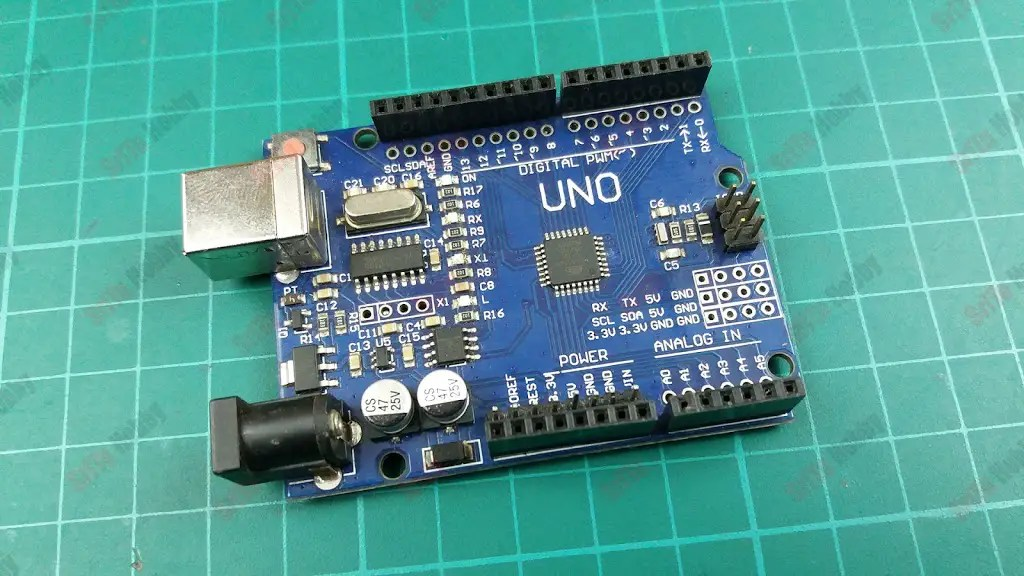#### Jumper wires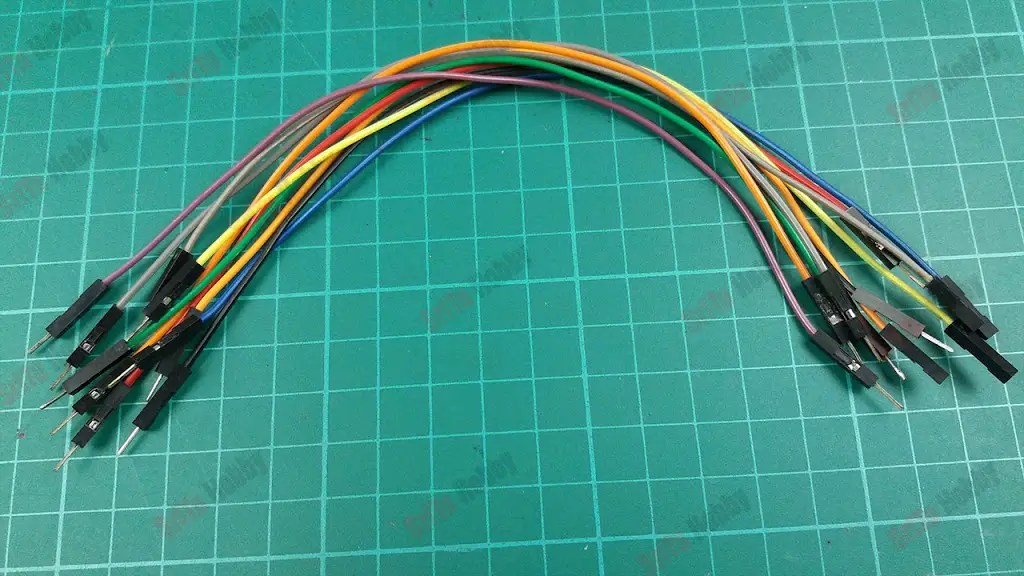### Step 2

Secondly, connect these components. For that, use the circuit diagram.Thirdly, let’s prepare the program for this project. It is as follows.  First, download and install the “Adafruit MPU6050” library.
The complete program of this project – Download
``````/*MPU6050 sensor with Arduino.
* http://srituhobby.com
*/

#include <LiquidCrystal_I2C.h>
#include <Wire.h>

LiquidCrystal_I2C lcd(0x27, 16, 2);

void setup(void) {
Serial.begin(115200);
lcd.init();
lcd.backlight();
mpu.begin();

mpu.setAccelerometerRange(MPU6050_RANGE_8_G);
mpu.setGyroRange(MPU6050_RANGE_500_DEG);
mpu.setFilterBandwidth(MPU6050_BAND_21_HZ);
delay(100);
}

void loop() {
sensors_event_t a, g, temp;
mpu.getEvent(&a, &g, &temp);

/* Print out the values */
Serial.print("Acceleration X: ");
Serial.print(a.acceleration.x);
Serial.print(", Y: ");
Serial.print(a.acceleration.y);
Serial.print(", Z: ");
Serial.print(a.acceleration.z);
Serial.println(" m/s^2");

Serial.print("Rotation X: ");
lcd.setCursor(0, 0);
lcd.print("R:");
lcd.print(g.gyro.x);
Serial.print(g.gyro.x);

Serial.print(", Y: ");
lcd.setCursor(0, 1);
lcd.print("P:");
lcd.print(g.gyro.y);
Serial.print(g.gyro.y);

Serial.print(", Z: ");
lcd.setCursor(8, 0);
lcd.print("Y:");
lcd.print(g.gyro.z);
Serial.print(g.gyro.z);

Serial.print("Temperature: ");
lcd.setCursor(8, 1);
lcd.print("T:");
lcd.print(temp.temperature);
Serial.print(temp.temperature);
Serial.println(" degC");

Serial.println("");
delay(100);
}``````

#### Code explanation

First, MPU6050, I2C, adafruit sensor, and wire libraries included.
#include <LiquidCrystal_I2C.h>
#include <Wire.h>

Then, creates an object for the MPU6050 library as “mpu”. Also, creates an object for the I2C library that includes the I2C address and the LCD length and width.
LiquidCrystal_I2C lcd(0x27, 16, 2);

In the setup function, the serial monitor, LCD, and sensor initialized.  Also, accelerometers, gyroscopes, and bandwidth ranges are set.
void setup(void) {
Serial.begin(115200);
lcd.init();
lcd.backlight();
mpu.begin();

Accelerometer range set. You can use the values 2,4,8 and 16 for this.
mpu.setAccelerometerRange(MPU6050_RANGE_8_G);

Gyroscope range set. You can use the 250,500,1000 and 2000 for this.
mpu.setGyroRange(MPU6050_RANGE_500_DEG);

Bandwidth set.
mpu.setFilterBandwidth(MPU6050_BAND_21_HZ);
delay(100);
}
In the loop function, gets the accelerometers, gyroscopes, and temperature values. After, printed on the serial monitor. Also, the gyroscope rotation values and temperature values are printed on the LCD.
void loop() {
sensors_event_t a, g, temp;
mpu.getEvent(&a, &g, &temp);

/* Print out the values */
Accelerations (X,Y,Z)
Serial.print(“Acceleration X: “);
Serial.print(a.acceleration.x);
Serial.print(“, Y: “);
Serial.print(a.acceleration.y);
Serial.print(“, Z: “);
Serial.print(a.acceleration.z);
Serial.println(” m/s^2″);
Rotetion (Roll, pitch and yaw)
Serial.print(“Rotation X: “);
lcd.setCursor(0, 0);
lcd.print(“R:”);
lcd.print(g.gyro.x);
Serial.print(g.gyro.x);

Serial.print(“, Y: “);
lcd.setCursor(0, 1);
lcd.print(“P:”);
lcd.print(g.gyro.y);
Serial.print(g.gyro.y);

Serial.print(“, Z: “);
lcd.setCursor(8, 0);
lcd.print(“Y:”);
lcd.print(g.gyro.z);
Serial.print(g.gyro.z);

Temperature scale.
Serial.print(“Temperature: “);
lcd.setCursor(8, 1);
lcd.print(“T:”);
lcd.print(temp.temperature);
Serial.print(temp.temperature);
Serial.println(” degC”);

Serial.println(“”);
delay(100);
}
Step 4
OK, now select board and port. Afterward, upload this code.